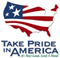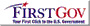Texas Water Science Center

# Statewide Analysis of the Drainage-Area Ratio Method for 34 Streamflow Percentile Ranges in Texas

By William H. Asquith, Meghan C. Roussel, and Joseph Vrabel

U.S. Geological Survey
Scientific Investigations Report 2006–5286

In cooperation with the Texas Commission on Environmental Quality

## Abstract

The drainage-area ratio method commonly is used to estimate streamflow for sites where no streamflow data are available using data from one or more nearby streamflow-gaging stations. The method is intuitive and straightforward to implement and is in widespread use by analysts and managers of surface-water resources. The method equates the ratio of streamflow at two stream locations to the ratio of the respective drainage areas. In practice, unity often is assumed as the exponent on the drainage-area ratio, and unity also is assumed as a multiplicative bias correction. These two assumptions are evaluated in this investigation through statewide analysis of daily mean streamflow in Texas. The investigation was made by the U.S. Geological Survey in cooperation with the Texas Commission on Environmental Quality. More than 7.8 million values of daily mean streamflow for 712 U.S. Geological Survey streamflow-gaging stations in Texas were analyzed. To account for the influence of streamflow probability on the drainage–area ratio method, 34 percentile ranges were considered. The 34 ranges are the 4 quartiles (0–25, 25–50, 50–75, and 75–100 percent), the 5 intervals of the lower tail of the streamflow distribution (0–1, 1–2, 2–3, 3–4, and 4–5 percent), the 20 quintiles of the 4 quartiles (0–5, 5–10, 10–15, 15–20, 20–25, 25–30, 30–35, 35–40, 40–45, 45–50, 50–55, 55–60, 60–65, 65–70, 70–75, 75–80, 80–85, 85–90, 90–95, and 95–100 percent), and the 5 intervals of the upper tail of the streamflow distribution (95–96, 96–97, 97–98, 98–99 and 99–100 percent). For each of the 253,116 (712X711/2) unique pairings of stations and for each of the 34 percentile ranges, the concurrent daily mean streamflow values available for the two stations provided for station–pair application of the drainage–area ratio method. For each station pair, specific statistical summarization (median, mean, and standard deviation) of both the exponent and bias–correction components of the drainage–area ratio method were computed. Statewide statistics (median, mean, and standard deviation) of the station–pair specific statistics subsequently were computed and are tabulated herein. A separate analysis considered conditioning station pairs to those stations within 100 miles of each other and with the absolute value of the logarithm (base–10) of the ratio of the drainage areas greater than or equal to 0.25. Statewide statistics of the conditional station-pair specific statistics were computed and are tabulated. The conditional analysis is preferable because of the anticipation that small separation distances reflect similar hydrologic conditions and the observation of large variation in exponent estimates for similar-sized drainage areas. The conditional analysis determined that the exponent is about 0.89 for streamflow percentiles from 0 to about 50 percent, is about 0.92 for percentiles from about 50 to about 65 percent, and is about 0.93 for percentiles from about 65 to about 85 percent. The exponent decreases rapidly to about 0.70 for percentiles nearing 100 percent. The computation of the bias-correction factor is sensitive to the range analysis interval (range of streamflow percentile); however, evidence suggests that in practice the drainage-area method can be considered unbiased. Finally, for general application, suggested values of the exponent are tabulated for 54 percentiles of daily mean streamflow in Texas; when these values are used, the bias correction is unity.

Abstract

Introduction

Purpose and Scope

Previous Studies

Database

Mathematics of the Drainage-Area Ratio Method

Generalized Drainage-Area Ratio Method

Parameter Estimation for Generalized Drainage-Area Ratio Method

Example Calculations of Parameter Estimation

Statewide Analysis of the Drainage-Area Ratio Method in Texas

Data Analysis

Implementation of the Drainage-Area Ratio Method in Texas

Sensitivity of the Drainage-Area Ratio Method

Limitations of the Drainage-Area Ratio Method

Example Application of the Drainage-Area Ratio Method

Summary

References

Appendix 1—Summary of the Drainage-Area Ratio Method for Daily Mean Streamflow in Texas

## Figures

 1. Map showing locations of U.S. Geological Survey streamflow-gaging stations in Texas with at least 1 year of daily mean streamflow data 2. Example output from drainage-area ratio computation run for an arbitrary station pair and the 98–99 and 99–100 percentile ranges 3–6. Graphs showing relation between: 3. Separation distance and exponent of drainage-area ratio method for the fourth quartile (75–100 percentile range) of daily streamflow in Texas 4. Absolute value of logarithm of ratio of drainage areas and exponent of drainage-area ratio method for fourth quartile (75–100 percentile range) of daily streamflow in Texas for station pairs with separation distance less than or equal to 100 miles 5. Conditional exponents (f) of drainage-area ratio method and percentile of daily mean streamflow in Texas by streamflow-frequency width interval 6. Conditional bias-correction factors (K) of drainage-area ratio method and percentile of daily mean streamflow in Texas by streamflow-frequency width interval 7. Example application of the drainage-area ratio method

## Tables

 1 U.S. Geological Survey streamflow-gaging stations in Texas with at least 1 year of daily mean streamflow data 2 Example of parameter estimation for the generalized drainage-area ratio method 3 Summary statistics of drainage-area ratio method for each of the 34 percentile ranges of streamflow in Texas 4 Summary statistics of drainage-area ratio method conditional on separation distance and relative drainage area for each of the 34 percentile ranges of streamflow in Texas 5 Suggested exponents for the drainage-area ratio method in Texas

This document is available in Portable Document Format (PDF):  (67.3 MB)

To view this document, you need the Adobe Acrobat® Reader installed on your computer.
(A free copy of the Acrobat® Reader may be downloaded from Adobe Systems Incorporated.)U.S. Department of the Interior | U.S. Geological Survey
Persistent URL: https://pubs.water.usgs.gov/sir20065286
Page Contact Information: USGS Publishing Network
Page Last Modified: Thursday, 01-Dec-2016 19:32:48 EST